# C++ Programs to Check Given Number is Palindrome or not

In this tutorial you will learn about the C++ Programs to Check Given Number is Palindrome or not and its application with practical example.

## C++ Programs to Check Given Number is Palindrome or not

In this tutorial, we will learn to create a C++ program that will Check Given Number is Palindrome or not using C++ programming.

## Prerequisites

Before starting with this tutorial we assume that you are best aware of the following C++ programming topics:

• Operators in C++ Programming.
• Basic Input and Output function in C++ Programming.
• Basic C++ programming.
• For loop in C++ programming.
• Conditional Statements in C++ programming.
• Arithmetic operations in C++ Programming.

## What is a palindrome number?

The Palindrome number means a number that is the same when we read it from forward and reverse.

For example:-

1. 1221 this is a palindrome number is the same when we write it in reverse order 1221.
2. 123 this is not a palindrome number it is not the same when we write it in reverse order 321.

## Program to Check Whether a Number is Palindrome or Not:-

To check whether a number is a Palindrome Number or not. In today’s C++ program we will write code for checking. First, we will take a number in input and then pass it into the program to Check Whether a Number is Palindrome or Not.

With the help of the below program, we can Check Palindrome or Not.

## Output:-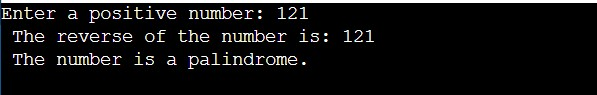In the above program, we have first initialized the required variable.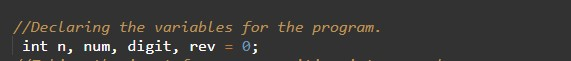• num= it will hold the integer value of the input.
• n = it will hold the integer value.
• digit = it will hold the integer value.
• rev = it will hold the integer value.

Input message for the user for the integer value.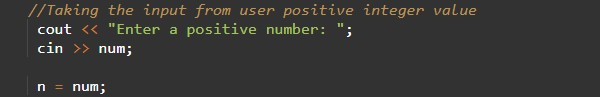Program Logic Code.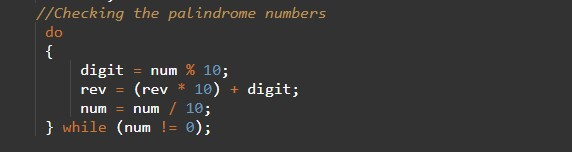Printing output Palindrome number or not.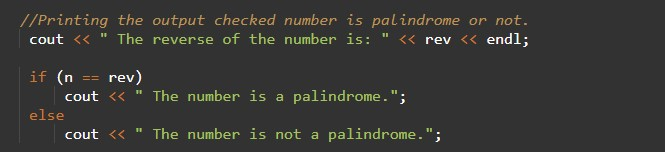In this tutorial we have learn about the C++ Programs to Check Given Number is Palindrome or not and its application with practical example. I hope you will like this tutorial.